# Failure Discounting

 Appendix A Failure Discounting

During a reliability growth test, once a failure has been analyzed and corrective actions for that specific failure mode have been implemented, the probability of its recurrence is diminished, as given in Lloyd . Then for the success/failure data that follow, the value of the failure for which corrective actions have already been implemented should be subtracted from the total number of failures. However, certain questions arise, such as to what extent should the failure value be diminished or discounted, and how should the failure value be defined? One answer would be to use engineering judgment (e.g., a panel of specialists would agree that the probability of failure has been reduced by 50% or 90% and therefore, that failure should be given a value of 0.5 or 0.9). The obvious disadvantage of this approach is its arbitrariness and the difficulty of reaching an agreement. Therefore, a statistical basis needs to be selected, one that is repeatable and less arbitrary. Failure discounting is applied when using the Lloyd-Lipow, logistic, and the standard and modified Gompertz models.

The value of the failure,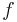$f\,\!$, is chosen to be the upper confidence limit on the probability of failure based on the number of successful tests following implementation of the corrective action. The failure value is given by the following equation: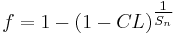$f=1-{{(1-CL)}^{\tfrac{1}{{{S}_{n}}}}}\,\!$

where:

•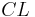$CL\,\!$ is the confidence level.
•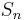${{S}_{n}}\,\!$ is the number of successful tests after the first success following the corrective action.

For example, after one successful test following a corrective action,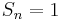${{S}_{n}}=1\,\!$, the failure is given a value of 0.9 based on a 90% confidence level. After two successful tests,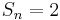${{S}_{n}}=2\,\!$, the failure is given a value of 0.684, and so on. The procedure for applying this method is illustrated in the next example.

Example

Assume that during the 22 launches given in the first table below, the first failure was caused by Mode 1, the second and fourth failures were caused by Mode 2, the third and fifth failures were caused by Mode 3, the sixth failure was caused by Mode 4 and the seventh failure was caused by Mode 5.

1. Find the standard Gompertz reliability growth curve using the results of the first 15 launches.
2. Find the predicted reliability after launch 22.
3. Calculate the reliability after launch 22 based on the full data set from the second table, and compare it with the estimate obtained for question 2.
Launch Number Result/Mode Failure 1 Failure 2 Failure 3 Failure 4 Failure 5 Sum of Failures Launch Sequence with Failure Modes and Failure Values 1 F1 1.000 1.000 2 F2 1.000 1.000 2.000 3 F3 0.900 1.000 1.000 2.900 4 S 0.684 0.900 1.000 2.584 5 F2 0.536 1.000 0.900 2.436 6 F3 0.438 1.000 1.000 2.438 7 S 0.369 0.900 1.000 2.269 8 S 0.319 0.684 0.900 1.902 9 S 0.280 0.536 0.684 1.500 10 S 0.250 0.438 0.536 1.224 11 S 0.226 0.369 0.438 1.032 12 S 0.206 0.319 0.369 0.894 13 S 0.189 0.280 0.319 0.788 14 S 0.175 0.250 0.280 0.705 15 S 0.162 0.226 0.250 0.638 16 S 0.152 0.206 0.226 0.584 17 F4 0.142 0.189 0.206 1.000 1.537 18 S 0.134 0.175 0.189 1.000 1.498 19 F5 0.127 0.162 0.175 0.900 1.000 2.364 20 S 0.120 0.152 0.162 0.684 1.000 2.118 21 S 0.114 0.142 0.152 0.536 0.900 1.844 22 S 0.109 0.134 0.142 0.438 0.684 1.507

Launch Number Calculated Reliability (%) ln(R) Gompertz Reliability (%) Comparison of the Predicted Reliability with the Actual Data 1 0.000 2 0.000 3 3.333 1.204 4 35.406 3.567 28.617 5 51.283 3.937 45.883 6 59.372 4.084 60.841 7 67.585 4.213 72.017 8 76.219 4.334 79.654 9 83.334 4.423 84.600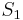${{S}_{1}}\,\!$ = 24.558 10 87.764 4.475 87.701 11 90.614 4.507 89.609 12 92.555 4.528 90.769 13 93.939 4.543 91.469 14 94.964 4.553 91.891 15 95.746 4.562 92.143${{S}_{2}}\,\!$ = 27.167 16 96.356 4.568 92.295 17 90.960 4.510 92.385 18 91.681 4.518 92.439 19 87.560 4.472 92.472 20 89.411 4.493 92.491 21 91.219 4.513 92.503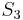${{S}_{3}}\,\!$ = 27.076 22 93.152 4.534 92.510

Solution

1. In the table above, the failures are represented by columns "Failure 1", "Failure 2", etc. The "Result/Mode" column shows whether each launch is a failure (indicated by the failure modes F1, F2, etc.) or a success (S). The values of failure are based on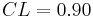$CL=0.90\,\!$ and are calculated from:$f=1-{{(1-CL)}^{\tfrac{1}{{{S}_{n}}}}}\,\!$
These values are summed and the reliability is calculated from: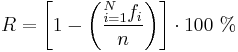$R=\left[ 1-\left( \frac{\mathop{}_{i=1}^{N}{{f}_{i}}}{n} \right) \right]\cdot 100\text{ }%\,\!$
where$N\,\!$ is the number of failures and$n\,\!$ is the number of events, tests, runs or launches.
• Failure 1 is Mode 1; it occurs at launch 1 and it does not recur throughout the process. So at launch 3,${{S}_{n}}=1\,\!$, and so on.
• Failure 2 is Mode 2; it occurs at launch 2 and it recurs at launch 5. Therefore,${{S}_{n}}=1\,\!$ at launch 4 and at launch 7, and so on.
• Failure 3 is Mode 3; it occurs at launch 3 and it recurs at launch 6. Therefore,${{S}_{n}}=1\,\!$ at launch 5 and at launch 8, and so on.
• Failure 6 is Mode 4; it occurs at launch 17 and it does not recur throughout the process. So at launch 19,${{S}_{n}}=1\,\!$, and so on.
• Failure 7 is Mode 5; it occurs at launch 19 and it does not recur throughout the process. So at launch 21,${{S}_{n}}=1\,\!$, and so on.
For launch 3 and failure 1,${{S}_{n}}=1\,\!$.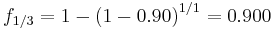\begin{align} {{f}_{1/3}}=1-{{(1-0.90)}^{1/1}}=0.900 \end{align}\,\!
For launch 4 and failure 1,${{S}_{n}}=2\,\!$.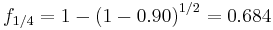\begin{align} {{f}_{1/4}}=1-{{(1-0.90)}^{1/2}}=0.684 \end{align}\,\!
And so on. Calculate the initial values of the Gompertz parameters using the second table above. Based on the equations from the Gompertz Models chapter, the initial values are:\begin{align} c &= \left ( \frac{S_{2}-S_{3}}{S_{2}-S_{1}} \right )^\frac{1}{n\cdot I} \\ &= \left [ \frac{27.167-27.076}{27.167-24.558} \right ]^\frac{1}{6} \\ &= 0.572 \\ \end{align}\,\!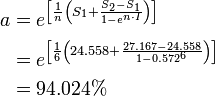\begin{align} a &= e^\left [\frac{1}{n}\left (S_{1} + \frac {S_{2}-S_{1}}{1-e^{n\cdot I}} \right )\right ] \\ &= e^\left [\frac{1}{6}\left (24.558 + \frac{27.167 - 24.558}{1-0.572^{6}}\right ) \right ] \\ &= 94.024% \\ \end{align}\,\!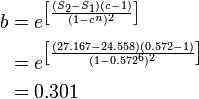\begin{align} b &= e^\left [\frac{(S_{2}-S_{1})(c-1)}{(1-c^{n})^{2}} \right ] \\ &= e^\left [\frac{(27.167-24.558)(0.572-1)}{(1-0.572^{6})^{2}} \right ] \\ &= 0.301 \\ \end{align}\,\!
Now, since the initial values have been determined, the Gauss-Newton method can be used. Substituting${{Y}_{i}}={{R}_{i}},\,\!$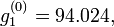$g_{1}^{(0)}=94.024,\,\!$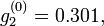$g_{2}^{(0)}=0.301,\,\!$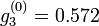$g_{3}^{(0)}=0.572\,\!$. The iterations are continued to solve for the parameters. Using the RGA software, the estimators of the parameters for the given example are: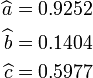\begin{align} \widehat{a}&= 0.9252 \\ \widehat{b} &= 0.1404 \\ \widehat{c} &= 0.5977 \end{align}\,\!
The next figure shows the entered data and the estimated parameters.

The Gompertz reliability growth curve may now be written as follows where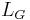${{L}_{G}}\,\!$ is the number of launches, with the first successful launch being counted as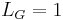${{L}_{G}}=1\,\!$. Therefore,${{L}_{G}}\,\!$ is equal to 19, since reliability growth starts with launch 4.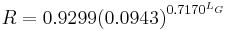$R=0.9299{{(0.0943)}^{{{0.7170}^{{{L}_{G}}}}}}\,\!$
2. The predicted reliability after launch 22 is therefore: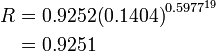\begin{align} R &= 0.9252{{(0.1404)}^{{{0.5977}^{19}}}} \\ &= 0.9251 \end{align}\,\!
The predicted reliability after launch 22 is calculated using the Quick Calculation Pad (QCP), as shown next.
3. In the second table, the predicted reliability values are compared with the reliabilities that are calculated from the raw data using failure discounting. It can be seen in the table, and in the following figure, that the Gompertz curve appears to provide a good fit to the actual data.